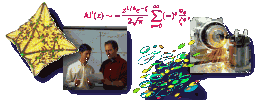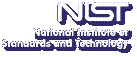Up# Critical features of the minimizers of non-smooth cost-functions; application to image processing.

Mila Nikolova
CNRS URA820 ENST Dpt. TSI, Paris, France

Friday, March 8, 2002 15:00-16:00,
Room 145, NIST North (820)
Gaithersburg
Friday, March 8, 2002 13:00-14:00,
Room 4550
Boulder

Abstract: We address applications where a sought vector x (an image, a signal) is recovered from data y by minimizing a cost-function f(x,y)=D(x,y)+R(x), where D is a data fidelity term and R is a regularization term. Our goal is to exhibit how the shapes of the functions D and R determine the essential features exhibited by the minimizers of f(.,y). Typically, the regularization term R is defined over the differences between neighboring samples of x. We show that when R is non-smooth, the minimizers of finvolve large regions where the differences between neighbors are null. This explains in particular the stair-casing effect observed in total variation methods. This striking property cannot be exhibited by the minimizers of smooth cost-functions. The data-fidelity term D comes from a statistical modelling of the data-acquisition and is usually a smooth function (and often quadratic). We showthat if D is non-smooth at the origin, typical data y lead to minimizers x of f(.,y) which fit exactly part of the data entries, i.e. they satisfy exactly a certain number of the data-fidelity equations. This surprising property does notoccur if D is smooth. An astute use of the properties explained above helps to conceive cost-functionswhose minimizers exhibit some special properties. E.g., we focus on the reconstruction of binary images where the main difficulty comes from the non-convexity of the relevant cost-functions. We show how to construct continuous-valued, convex cost-functions whose minimizers are quasi-binary (theyinvolve only very few non-binary pixels). Next, we exhibit how to process outliers, or spiky images, by using non-smooth data-fidelity terms. Numerical experiments will be shown for all these applications.
Contact: A. S. Carasso

Note: Visitors from outside NIST must contact Robin Bickel; (301) 975-3668; at least 24 hours in advance.